SCERT AP 7th Class Maths Solutions Pdf Chapter 1 Integers InText Questions and Answers.

## AP State Syllabus 7th Class Maths Solutions 1st Lesson Integers InText Questions

Fill in the blanks: (Page No: 3)

Question 1.
7 × (-4) = – (7 × 4) = ___________
– 28

Question 2.
2 × (-6) = – (2 × 6) = ___________
– 12Check Your Progress (Page No: 4)

Find the values of

(i) 4×(-8)
4 × (-8) = -(4×8)
= – 32

(ii) 5 × (-20)
5 × (-20) = – (5 × 20)
= – 100

(iii) 7 × (-8)
7 × (-8) = – (7 × 8)
= – 56

(iv) 10 × (-9)
10 × (- 9) = -(10 × 9)
= – 90

Fill in the blanks: (Page No: 4)

Question 1.
– 3 × 4 = ________ = 3 × (-4)
– 12

Question 2.
– 4 × 4 = ________ = 4 × (-4)
– 16Let’s Explore: (Page No: 4)

Question 1.
Prepare a pattern to find (- 3) × 5 starting from 4 × 5.
4 × 5 = 20
3 × 5 = 15
2 × 5 = 10
1 × 5 = 5
0 × 5 = 0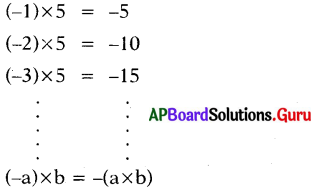Question 2.
Prepare a pattern to find (- 7) × 3 starting from 5 × 3.
5 × 3 = 15
4 × 3 = 12
3 × 3 = 9
2 × 3 = 6
1 × 3 = 3
0 × 3 = 0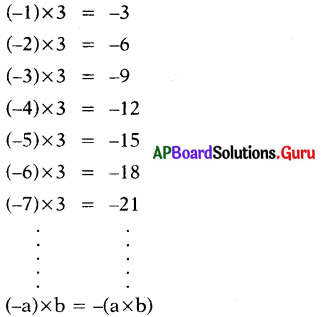Find the values of (Page No: 4)

Question 1.
(-6) × 7 = __________ = -(6 × 7) = – 42
6 × (-7)

Question 2.
(-2) × 5 = __________ = -(2 × 5) = – 10
2 × (-5)Question 3.
(-3) × 6 = __________ = -(3 × 6) = – 18
3 × (-6)

Question 4.
(-4) × 5 = __________ = -(4 × 5) = – 20
4 × (-5)

Check Your Progress (Page No. 4)

Find the values of

(i) (-6) × 5
(- 6) × 5 = -(6 × 5) = – 30

(ii) (- 15) × 2
(- 15) × 5 = – (15 × 2) = – 30

(iii) (- 12) × 8
(- 12) × 8 = – (12 × 8) = – 96

(iv) (- 10) × 6
(- 10) × 6 = – (10 × 6) = – 60

Find the values of (Page No: 4)

Question 1.
(- 2) × (- 4) = _________
8

Question 2.
– 2 × (- 5) = _________
10Check Your Progress (Page No: 5)

Question 1.
Prepare a pattern to find (-5) × (-4) starting from (-5) × 3.
-5 × 3 = – 15
-5 × 2 = – 10
-5 × 1 = – 5
-5 × 0 = 0
-5 × (-1) = + 5
-5 × (-2) = + 10
-5 × (-3) = + 15
-5 × (-4) = + 20

Question 2.
Prepare a pattern to find (- 7) × (- 2) starting from (- 7) × 5.
– 7 × 5 = – 35
– 7 × 4 = – 28
– 7 × 3 = – 21
– 7 × 2 = – 14
– 7 × 1 = – 7
– 7 × 0 = 0
– 7 × (- 1) = + 7
– 7 × (- 2) = + 14

Let’s Do Activity (Page No: 6)

Fill the grid by multiplying each number in the first column with each number in the hirst row and answer the following questions.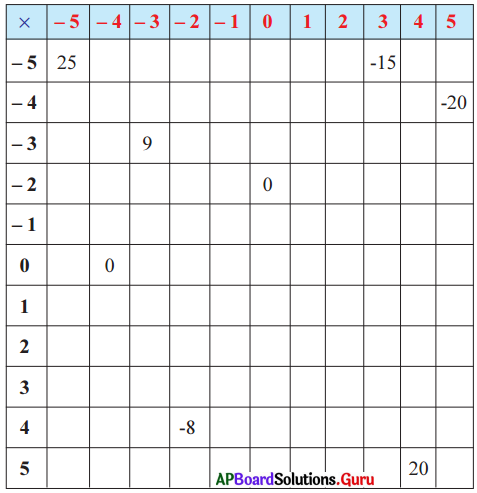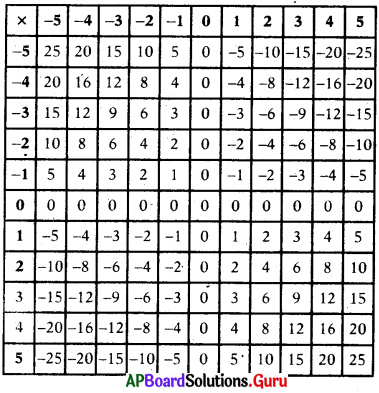Question 1.
Write your observations from the table.
Product of two integers with same sign is always a positive integer and the product of two integers with opposite signs is always negative integer.

Question 2.
What happens when an integer multiplied with (-1) ?
a × (-1) = – 1 × a = – a.
When an integer is multiplied by – 1, the sign of the integer changes from + ve to – ve or – ve to + ve.

Question 3.
When will we get product of two integers is zero ?
The product of two integers is zero only when one of them is zero.

Division of Integers (Page No: 8)

Observe the following and fill the blanks

 Multiplication Statements Division Statements 5 × 3 = 15 15 ÷ 3 = 5 15 ÷ 5 = 3 6 × (-2) = – 12 (-12) ÷ 6 = ___________ (-12) ÷ (-2) = ___________ (-10) × 2 = -20 (-20) ÷ (-10) = ___________ ______________________ (-5) × (-6) = 30 ______________________ ______________________

 Multiplication Statements Division Statements 5 × 3 = 15 15 ÷ 3 = 5 15 ÷ 5 = 3 6 × (-2) = – 12 (-12) ÷ 6 = (-2) (-12) ÷ (-2) = 6 (-10) × 2 = -20 (-20) ÷ (-10) = 2 (-20) ÷ 2 = (-10) (-5) × (-6) = 30 30 ÷ (-6) = (-5) 30 ÷ (-5) = (-6)

Check Your Progress (Page No: 9)

Fill the following table.

 Integer 1 ÷ Integer 2 Quotient 1. +(25) ÷ (+5) 5 2. 42 ÷ (-6) 3. (-75) ÷ 15 4. (-27) ÷ (-3)

 Integer 1 ÷ Integer 2 Quotient 1. +(25) ÷ (+5) 5 2. 42 ÷ (-6) (-7) 3. (-75) ÷ 15 (-5) 4. (-27) ÷ (-3) 9Let’s Do Activity (Page No: 10)

The fish in the pond below, carry some numbers. Choose any 4 pairs and carry out four multiplications with those numbers. Now, choose four other pairs and carry out divisions with those numbers.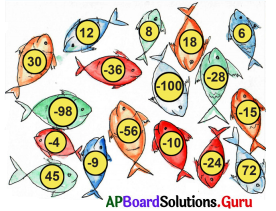Multiplications:
(i) (- 10) × 6 = – 60
(ii) (- 4) × (- 9) = 36
(iii) 12 × 6 = 72
(iv) 6 × (-4) = – 24
(v) 8 × 6 = 48
(vi) 12 × 8 = 96

Divisions:
(i) (-36) ÷ 6 = – 6
(ii) (-100) ÷ (- 10) = 10
(iii) 45 ÷ (-9) = – 5
(iv) 72 ÷ 12 = 6
(v) (-56) ÷ 8 = – 7
(vi) (-28) ÷ (-4) = 7

Puzzle time (Page No: 11)

Jasvi says her favorite number through puzzle. Find it.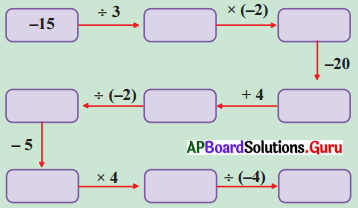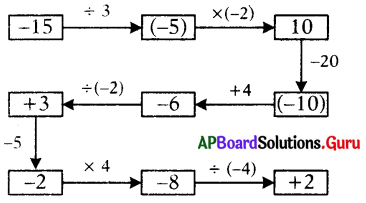(i) Closure property: Observe the following tables and complete them.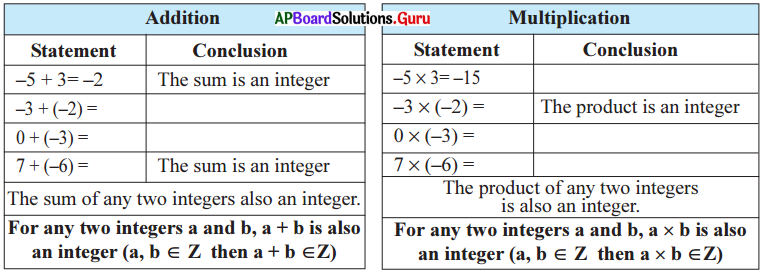∴ Integers are closed under addition and multiplication.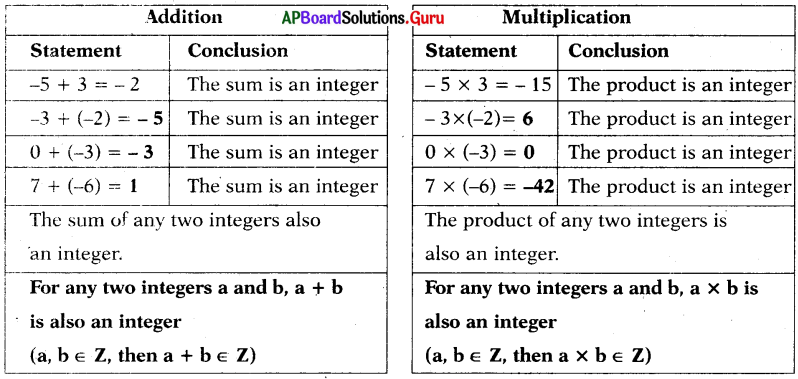∴ Integers are closed under addition and multiplication.

Let’s Think (Page No: 11)

Can you find at least one pair of integers whose sum or product is not an integer ?
Sol.
No, we can’t find at least one pair of integers whose sum of product is not an integer.

Page No. 12

(i) Closure property: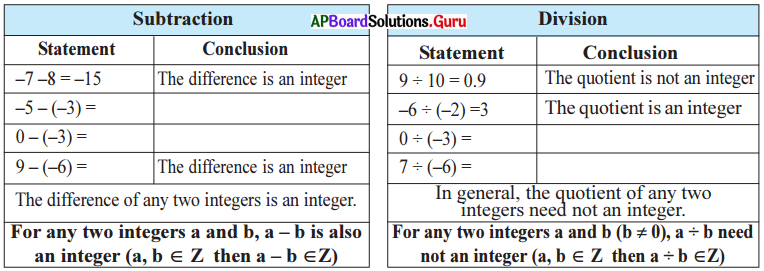∴ Integers are closed under subtraction, but need not closed under division.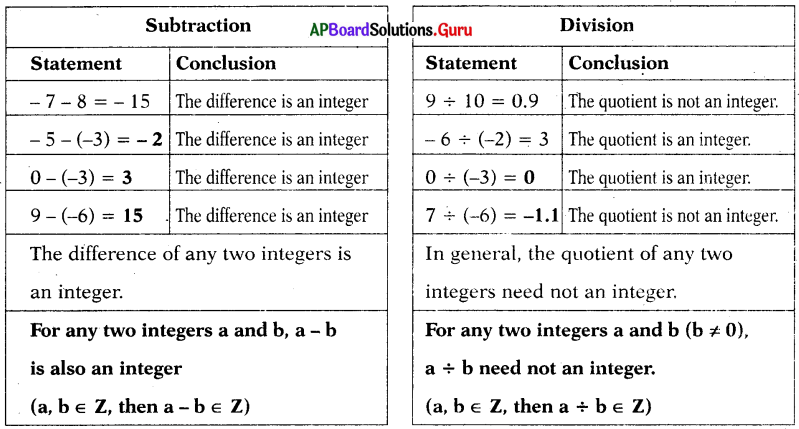∴ Integers are closed under subtraction, but need not closed under division.(ii) Commutative Law: Observe the following tables and complete them.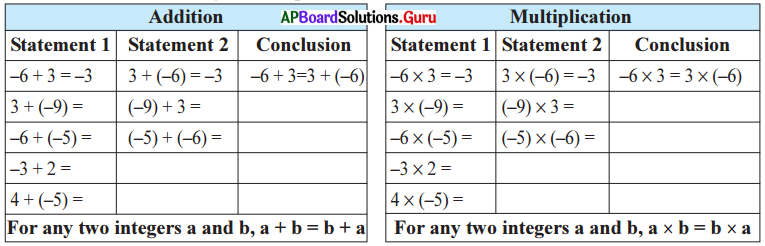∴ Integers are commutative under addition and multiplication.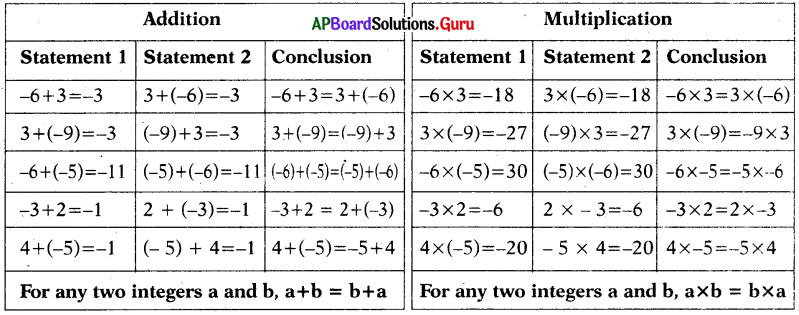∴ Integers are commutative under addition and multiplication.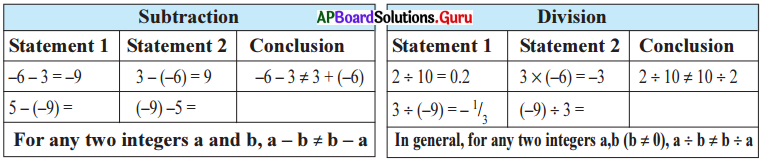∴ Integers are not commutative under subtraction and division.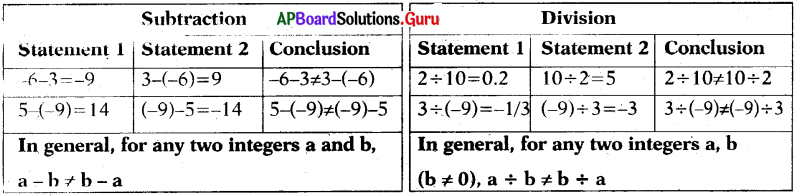∴ Integers are not commutative under subtraction and division.

Page No. 13

(iii) Associative Law: Observe the following tables and complete them.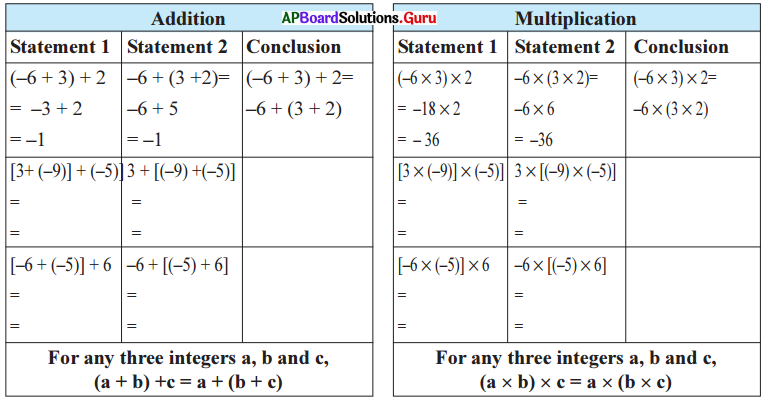∴ Integers are associative under addition and multiplication.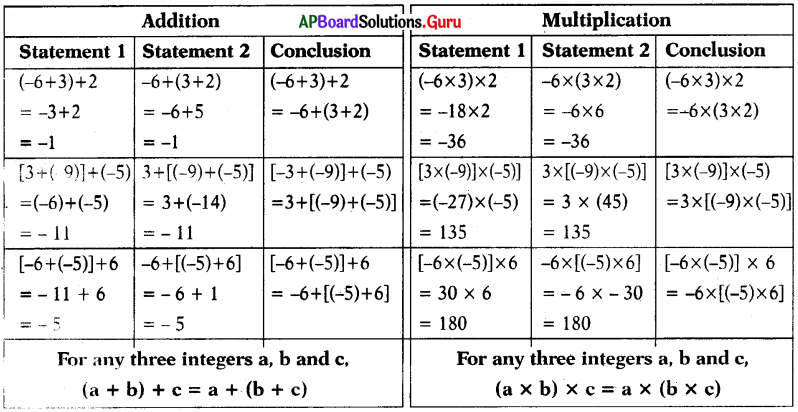∴ Integers are associative under addition and multiplication.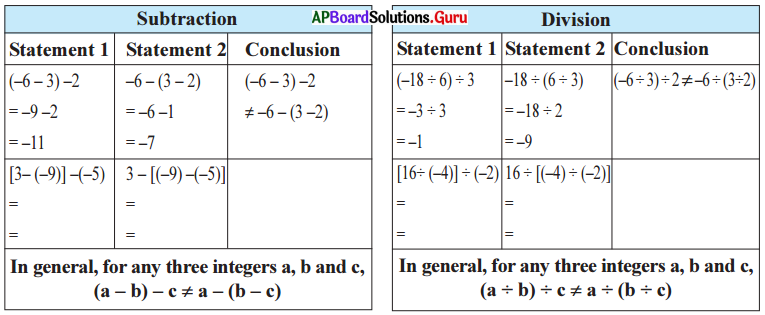∴ Integers are associative under subtraction and division.iv) Identity property: Observe the following tables and complete them.

 Addition Multiplication 3 + _______ = 3 3×1=3 0 + (- 3) = _______ _______ × (- 3) = – 3 -2 + _______ = – 2 -2× _______ = -2 _______ + 5 = 5 _______ ×5=5 -6+_______ =-6 -6×_______ =-6 For any integer a, a + 0 = 0 + a = a For any integer a, a × 1 = 1 × a = a ∴ Zero is the additive identity. ∴ 1 is multiplicative identity.

 Addition Multiplication 3 + 0 = 3 3×1=3 0 + (- 3) = – 3 1 × (- 3) = – 3 -2 + 0 = – 2 -2×1 = -2 0 + 5 = 5 1 × 5 = 5 -6+0=-6 – 6 × 1 = – 6 For any integer a, a + 0 = 0 + a = a For any integer a, a × 1 = 1 × a = a ∴ Zero is the additive identity. ∴ 1 is multiplicative identity.

Page No: 14

v. What should be added to – 3 to get additive identity 0 ?

A. We add + 3 to get additive identity 0. Observe the following.
(i) 4 + (- 4) = _________
0

(ii) (-5) + 5 = _________
0

(iii) (- 6) + _________ = 0
6

Check Your Progress (Page No: 14)

Question 1.
Write the additive inverses of 5, -8, 1 and 0.

 Number Additive inverse 5 -5 -8 8 1 – 1 0 0

Lets Think (Page No: 14)

Question 1.
What should be multiplied by 6 to get multiplicative identity 1 ? Is it exist in integers ?
6 × $$\frac{1}{6}$$ = 1 (OR) 6 ÷ 6
But $$\frac{1}{6}$$ is not a integer.
Multiplicative identity of 6 is $$\frac{1}{6}$$.
∴ $$\frac{1}{6}$$ does not exist in integers.Question 2.
Verify – 3 × [(-4) – 2] = [(- 3) × (- 4)] – [(- 3) × 2]. Is-multiplication distribute over subtraction of integers ? Write your observations.
– 3 × [(- 4) – 2] = [(- 3) × (- 4)] – [(-3) × 2]
– 3 × [- 4 – 2] = [3 × 4] – [- (3 × 2)]
– 3 × (-6) = 12 – (-6)
3 × 6 = 12 + 6
18 = 18
L.H.S. = R.H.S.
Yes it is multiplication distribute over subtraction of integers.
That is a × (b – c) = (a × b) – (a × c)
Yes, it exists in integers.

Check Your Progress (Page No: 17)

Simplify the following.

(i) 5 × 6 – 6
5 × 6 – 6
= 30 – 6 (Multiplication)
= 24 (Subtraction)

(ii) 24 ÷ 3 × 3 – 30
24 ÷ 3 × 3 – 30 (Division)
= 8 × 3 – 30 (Multiplication)
= 24 – 30 (Subtraction)
= – 6

(iii) 5 × 5 – 5 ÷ 5 + 5
5 × 5 – 5 ÷ 5 + 5 (Division)
= 5 × 5 – 1 + 5 (Multiplication)
= 25 – 1 + 5 (Addition)
= 25 + 5 – 1
= 30 – 1 (Subtraction)
= 29Let’s Explore (Page No: 19)

Question 1.
If |x| = 15, then what will be the value of x ? Discuss.
Given |x| = 15
If x > 0, then |x| = + 15
If x < 0, then |x| = – 15
So, – 15 < x < 15

Examples

Question 1.
A sump is full of water, when the motor started pumping the level of water decreasing 2 inches per minute then what is the level of water from the ground level after 20 minutes ?
The change in level of water per minute = – 2 inches (decreasing 2 inches)
The level of water after 20 minutes = 20 × (-2) – 40 inches
So, the level of water in sump is 40 inches depth from the ground level.

Question 2.
An elevator begins from 20 m above the ground. It descends into a mine shaft at the rate of 6 m per minute. What will be its position after 15 minutes ?
Since the elevator is going down, so the distance covered by it will be represented by a negative integer. Change in position of the elevator in one minute = – 6 m.
Change in position of the elevator in 15 minutes = 15 × (-6) = – 90 m.
So, the final position of the elevator – 20 + (-90) = – 70 m.
The elevator is at 70 m below the ground level.

Question 3.
In a test, ( + 5) marks are given for every correct answer and (-3) marks are given for every incorrect answer. Lakshmi gets 45 correct and 15 in-correct answers. What is her score ?
Marks given for one correct answer = 5
Marks obtained for 45 correct answers = 45 × 5 = 225
Marks given for one incorrect answer = – 3
Marks obtained for 15 incorrect answers = 15 × (- 3) = – 45
∴ Lakshmi’s score = 225 + (- 45)
= 180.

Question 4.
A borewell machine drills down 72 feet per hour from surface of the earth. If the water is at 360 feet down from surface of earth, after how many hours it will touch the water layer ?Depth of drilling in one hour = – 72 feet
Depth of water layer from surface of earth = – 360 feet
Number of hours required
= – 360 ÷ (-72)
= 5
Hence, the borewell machine will touch water layer at 5 hours of drilling.Question 5.
In a test, (+ 4) marks are given for every correct answer and (- 2) marks are given for every incorrect answer. Sasi answered all the questions and scored 26 marks from 8 correct answers. How many incorrect answers had Sasi attempted?
Marks given for one correct answer = 4
So, marks given for 8 correct answers
= 4 × 8
= 32
Sasi score = 26
Marks obtained for incorrect answers = 26 – 32 = -6
Marks given for one incorrect answer = – 2
= (- 6) ÷ (- 2)
= 3

Question 6.
Shop keeper Yaseen earns a profit of ₹ 20 per bag of Sonamasoori rice sold and loss of ₹ 12 per bag of Hamsa rice. In one week he gets neither profit nor loss, if he solds 1440 Sonamasoori rice bags. How many Hamsa rice bags did he sell ?
In the given problem there is neither profit nor loss.
So, profit earned + loss incurred = 0
Profit earned = – loss incurred
Profit earned by selling one Sonamasoori rice bag = ₹ 20
Profit earned by selling 1440 Sonamasoori rice bags
= 1440 × 20
= ₹ 28800
Loss incurred by selling one Hamsa rice bag = ₹ 12,
which we denoted by – 12 Loss incurred by selling one Hamsa rice bags = ₹ – 28800
Total number of Hamsa rice bags sold
= (- 28800) ÷ (-12)
= ₹ 2400 bags.

Question 7.
Find the additive inverses of (+ 2) and (- 3).
Additive inverse of + 2 = – (+2) = – 2
Additive inverse of – 3 = – (-3) = + 3.

Question 8.
Multiply the following using associative law.
(i) – 25 × (- 4) × 2 × (- 8)
– 25 × (- 4) × 2 × (- 8)
= [- 25 × (- 4)] × 2 × (- 8)
= [100 × 2] × (-8)
= 200 × (-8) = – 1600(ii) (- 20) × (- 2) × (- 5) × 7
(- 20) × (-2) × (-5) × 7
= (- 20) × [(-2) × (-5)] × 7
= [(- 20) × 10] × 7
= – 200 × 7
= – 1400

Question 9.
Are (-42) × (-7) and (-7) × (-42) equal ? Which is this law ?
(-42) × (-7) = + 294
(-7) × (-42) = + 294
∴ (- 42) × (- 7) = (- 7) × (- 42)
It is multiplicative commutative law.

Question 10.
Simplify 26 × (- 48) + (- 48) × (- 36) using suitable laws.
26 × (- 48) + (- 48) × (- 36)
= (- 48) × 26 + (- 48) × (- 36) (Commutative law)
= (- 48) × [26 + (- 36)] (Distributive law)
= (- 48) × (- 10) = 480

Question 11.
Simplify: 3 × 2 + 8 ÷ 4
3 × 2 + 8 ÷ 4 (Division)
= 3 × 2 ÷ 2 (Multiplication)
= 8

Question 12.
Simplify : 7 × 6 – $$\overline{8-4}$$.
7 × 6 – $$\overline{8-4}$$ (Vinculum)
= 7 × 6 – 4 (Multiplication)
= 42 – 4 (Subtraction)
= 38Question 13.
Simplify: 18 + 64 ÷ 4 {26 – (14 – $$\overline{7-3}$$)}
18 + 64 ÷ 4 {26 – (14 – $$\overline{7-3}$$)}
= 18 + 64 ÷ 4 {26 – (14 – 4)} (Vinculum)
= 18 + 64 ÷ 4 {26 – (14 – 4)} (Simple bracket)
= 18 + 64 ÷ 4{26 – 10} (Curly bracket)
= 18 + 64 ÷ 4 {16} (Of)
= 18 + 64 ÷ 64 (Division)
= 19Practice Questions (Page No: 21)

Question 1.
12, 19, 26, 33, 40, 47,
(a) 57
(b) 54
(c) 52
(d) 50
(b) 54

Explaination:
Given 12, 19, 26, 33, 40, 47, __________
Each number in the series obtained by adding 7 to the previous number.
So, next number is 47 + 7 = 54.

Question 2.
2, 13, 24, 35, 46, 57, __________
(a) 65
(b) 67
(c) 68
(d) 72
(c) 68

Explaination:
Given 2, 13, 24, 35, 46, 57, _________
Each number in the series obtained by adding 11 to the previous number. So, next number is 57 + 11 = 68.

Question 3.
61,67.71,73,79.
(a) 89
(b) 87
(c) 85
(d) 83
(d) 83

Explaination:
Given 61, 67, 71, 73, 79, _________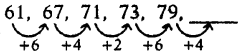So, answer is 79 + 4 = 83

Question 4.
3, 7, 13, 21, 31,
(a) 43
(b) 48
(c) 51
(d) 53
(a) 43Explaination:
Given 3, 7, 13, 21, 31, ___________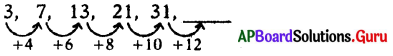So, answer is 31 + 12 = 43.

Question 5.
8, 12, 20, 32, 52, 84,
(a) 111
(b) 126
(c) 136
(d) 174
(c) 136

Explaination:
Given 8, 12, 20, 32, 52, 84, ___________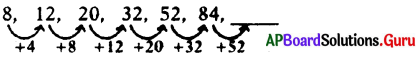So, answer is 84 + 52 = 136

Question 6.
23,28,38,53,73,98,
(a) 121
(b) 128
(c) 135
(d) 146
(b) 128

Explaination:
Given 23, 28, 38, 53, 73, 98, _______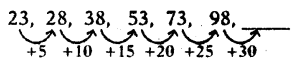So, answer is 98 + 30 = 128

Question 7.
101, 97, 89, 83, 79, 73, 71, ___________
(a) 61
(b) 65
(c) 66
(d) 67
(d) 67

Explaination:
Given 101, 97, 89, 83, 79, 73, 71, ________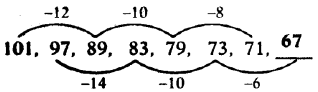So, answer is 73 – 6 = 67Question 8.
4, 7, 11, 18, 29, 47, _______
(a) 67
(b) 76
(c) 84
(d) 92
(b) 76

Explaination:
Given 4, 7, 11, 18, 29, 47, _______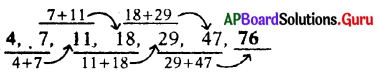So, answer is 29 + 47 = 76

Question 9.
76, 187, 298, 409, 520,
(a) 631
(b) 656
(c) 701
(d) 724
(a) 631

Explaination:
Given 76, 187, 298, 409, 520, _______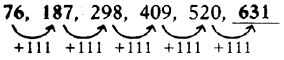So, answer is 520 + 111 = 631

Question 10.
0, 2, 5, 10, 17, 28, 41, ___________
(a) 50
(b) 53
(c) 57
(d) 58
(d) 58

Given 0, 2, 5, 10, 17, 28, 41, ______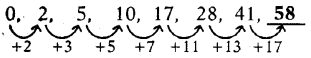2, 3, 5, 7, 11, 13, 17 all are prime numbers.
So, answer is 41 + 17 = 58

Question 11.
36, 45, 53, 60, 66, 71, ________
(a) 84
(b) 78
(c) 75
(d) 73
(c) 75

Explaination:
Given 36, 45, 53, 60, 66, 71, _______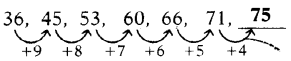So, the answer is 71 + 4 = 75.Question 12.
0, 15, 45, 90, 150, 225, _________
(a) 295
(b) 300
(c) 315
(d) 360
(c) 315

Explaination:
Given 0, 15, 45, 90, 150, 225, ___________
0, 0 + (15× 1), 15 + (15 × 2), 45 + (15 × 3), 90 + (15 × 4), 150 + (15 × 5), 225 + (15 × 6), 0, 0 + 15, 15 + 30, 45 + 45, 90 + 60, 150 + 75, 225 + 90
0, 15, 45, 90, 150, 225, 315
So, the answer is 225 + (15 × 6) = 315

Question 13.
18, 23, 25, 30, 32, 37, _______
(a) 43
(b) 41
(c) 39
(d) 38
(c) 39

Explaination:
Given 18, 23, 25, 30, 32, 37, ________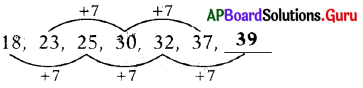So, the answer is 32 + 7 = 39

Question 14.
4, 7, 11, 18, 29, 47, _________
(a) 71
(b) 76
(c) 77
(d) 82
(b) 76

Explaination:
Given 4, 7, 11, 18, 29, 47, _______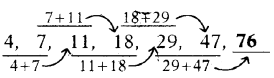So, the answer is 29 + 47 = 76Question 15.
12, 18, 21, 27, 30, 36, 39, ______
(a) 43
(b) 45
(c) 49
(d) 52